## Solid Density From Retort Analysis Mud Calculation

Retort analysis is the method to determine solid and liquid components in the drilling fluid. In this article, we will adapt mass balance and retort analysis data to determine solid density in the mud.Mass balance for mud is listed below;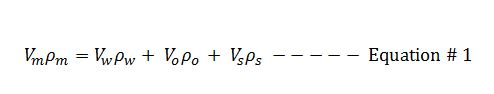We can rearrange the Euqation#1 in order to determine the solid density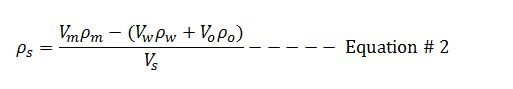## Coring Cost Per Footage Drilled

Coring is a special process to recover wellbore rock in the well.Figure 1 – Core from the well. Credit – Wikipedia

This article will demonstrate how to calculate coring cost per footage recovered.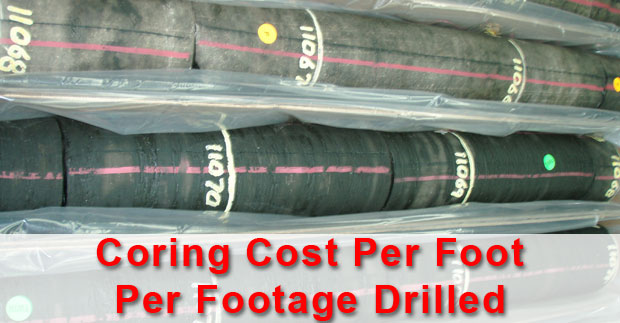Coring cost per footage recovered is expressed below;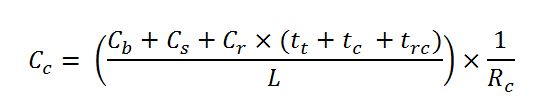## Volume of Cutting Generated While Drilling

While drilling, cuttings are generated every footage drilled and this topic will demonstrate how to determine volume of cutting entering into the wellbore.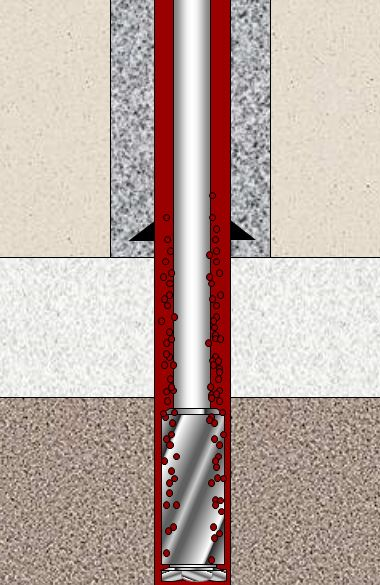Figure 1 – Cutting Generated While Drilling

The following formula is used to calculate cutting volume generated while drilling;Where;

Vc is volume of cutting in bbl/hr.

Ø is formation porosity (%).

D is wellbore diameter in inch.

ROP is rate of penetration in feet per hour.

Vc can be presented in several unit as follows;

## Buoyancy Factor with Two Different Fluid Weights in The WellBuoyancy Factor is the factor that is used to compensate loss of weight due to immersion in drilling fluid and you can find more information from this article > buoyancy factor calculation .  In that article, it demonstrates the buoyancy formula only for one fluid in the wellbore. However, this time, we will have the details about buoyancy factor when inside and outside fluid are different.

Buoyancy factor with different fluid inside and outside of tubular is listed below;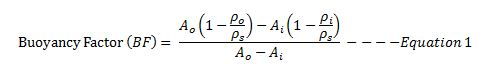Where;

Ao is an external area of the component.

Ai is an internal area of the component.

ρo is fluid density in the annulus at the component depth in the wellbore.

ρi is fluid density in the component depth in the wellbore.

ρs is steel weight density. Steel density is 65.4 ppg.

If you can the same mud weight inside and outside, the equation 1 will be like this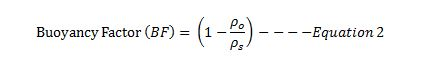Let’s take a look at the following example to get more understanding.

Example

13-3/8” casing shoe was at 2,500’MD/2,000’TVD

9-5/8” casing was run to 6,800’MD/6,000 TVD.

9-5/8” casing weight is 40 ppf and casing ID is 8.835 inch.

Current mud weight is 9.5 ppg oil based mud.

The well bore diagram is show below (Figure 1).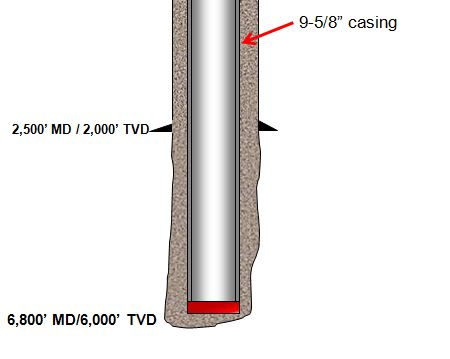Figure 1 – Wellbore Diagram

The well is planned to cement from shoe to surface and the planned cement weight is 14.0 ppg. The displacement fluid is drilling mud currently used.

• Air weight of casing string
• Buoyed weight of casing in drilling mud
• Buoyed weight of casing when cement is inside casing and drilling mud is outside casing
• Buoyed weight of casing when cement is outside casing and drilling mud is inside casing

Air weight of casing string

Air weight of casing string, lb = length of casing, ft × casing weight, lb/ft

Air weight of casing string, lb = 6,800 × 40 = 272,000 lb

Buoyed weight of casing in drilling mudFigure 2 – Buoyed Weight When Submersed In Drilling Mud

Buoyed weight = Buoyancy Factor (BF) × Air Weight of Casing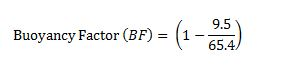Buoyancy Factor (BF) = 0.855

Buoyed weight = 0.855 × 272,000 = 232,489 lb

Buoyed weight of casing when cement is inside casing and drilling mud is outside casing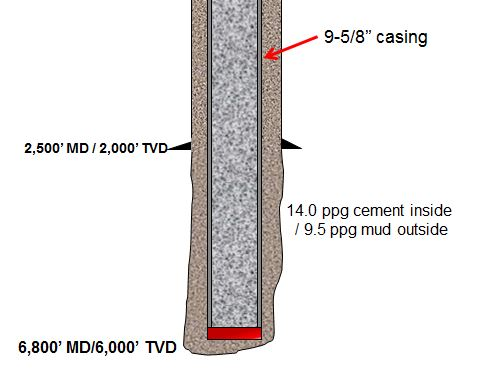Figure 3 – Buoyed weight of casing when cement is inside casing and drilling mud is outside casing

We will apply the Equation-1 for this case.

Ao is an external area of the component.

Ao = π × (Outside Diameter of casing)2 ÷ 4

Ao = π × (9.625)2 ÷ 4 = 72.76 square inch

Ai is an internal area of the component.

Ai = π × (Inside Diameter of casing)2 ÷ 4

Ai = π × (8.835)2 ÷ 4 = 61.31 square inch

ρo = 9.5 ppf (mud in the annulus)

ρi = 14.0 ppg (cement inside casing)

ρs = 65.4 ppg.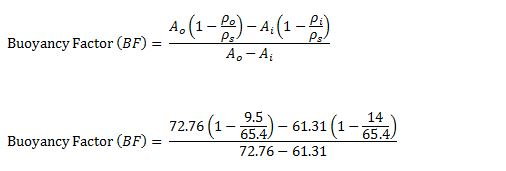Buoyancy Factor (BF) = 1.22

Buoyed weight = 1.22 × 272,000 = 331,840 lb

Buoyed weight of casing when cement is outside casing and drilling mud is inside casing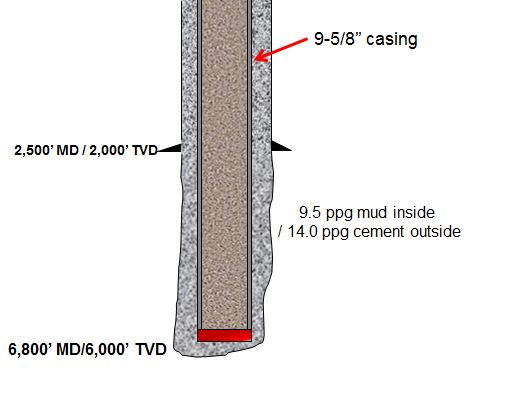Figure 4 – Buoyed weight of casing when cement is outside casing and drilling mud is inside casing

We will apply Equation-1 for this case as well.

All the calculation parameters are the same.

Ao = π × (9.625)2 ÷ 4 = 72.76 square inch

Ai = π × (8.835)2 ÷ 4 = 61.31 square inch

ρo = 9.5 ppg (mud in the annulus)

ρi = 14.0 ppg (cement inside casing)

ρs = 65.4 ppg.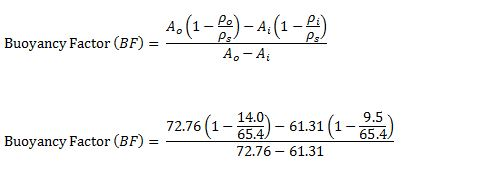Buoyancy Factor (BF) = 0.42

Buoyed weight = 0.42 × 272,000 = 114,240 lb

Conclusion: At different stage of the well, you may have different buoyed weight depending on density of fluid inside and outside of the component and it is not always that buoyed weight is less than air weight.

Reference book =>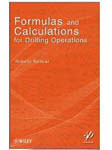Formulas and Calculations for Drilling Operations

## How does the 0.052 constant come from?

In the hydro-static pressure formula, if you use an oil field unit, you must see the hydro-static formula like this

Pressure, psi = 0.052 x Mud Weight (ppg) x TVD (ft)

I got the question asking about how the constant 0.052 is figured out.What is 0.052?

0.052 is a constant used for converting ppg-ft into pound per square inch (lb/in2)

Do you know how it comes from? Let’s get started

First of all, the unit conversions that you need to know are as follows:

1 gallon = 231 cubic inch (inch3)

1 ft = 12 inch

Convert density from ppg (pound per gallon) to lb per cubic inch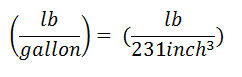Basic hydrostatic pressureThe constant can be determined by substituting the unit conversion.The constant is 0.051948 and if we round it up, it will be 0.052.